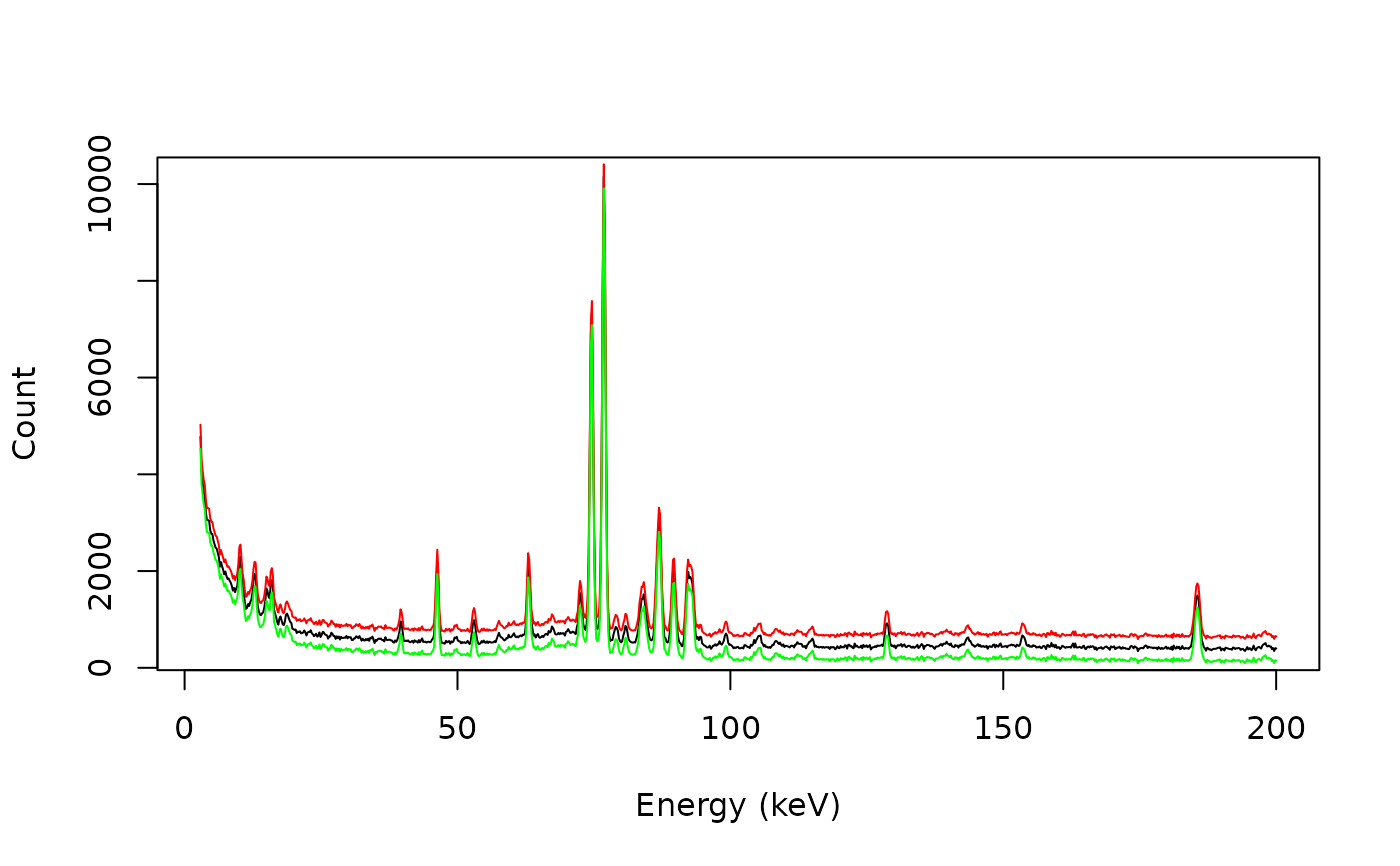Drift Intensities

## Usage

signal_drift(x, y, lag, ...)

# S4 method for numeric,numeric,numeric
signal_drift(x, y, lag)

# S4 method for ANY,missing,ANY
signal_drift(x, lag, subtract = FALSE)

## Arguments

x, y

A numeric vector. If y is missing, an attempt is made to interpret x in a suitable way (see grDevices::xy.coords()).

lag

A numeric vector specifying the offset or any object that can be interpreted in a suitable way (see grDevices::xy.coords())

...

Currently not used.

subtract

A logical scalar: should lag be subtracted to y?

## Value

Returns a list with two components x and y.

Other signal processing methods: signal_bind(), signal_correct(), signal_mean(), signal_shift(), subset()

N. Frerebeau

## Examples

## gamma-ray spectrometry
data("BEGe")

## Subset from 2.75 to 200 keV
BEGe <- signal_select(BEGe, from = 3, to = 200)

## Drift
BEGe_plus <- signal_drift(BEGe, lag = 250)
BEGe_minus <- signal_drift(BEGe, lag = 250, subtract = TRUE)

plot(BEGe, type = "l", xlab = "Energy (keV)", ylab = "Count")
lines(BEGe_plus, type = "l", col = "red")
lines(BEGe_minus, type = "l", col = "green")